SSC (English Medium) Class 9Maharashtra State Board
Share

# Balbharati solutions for Class 9 Geometry chapter 3 - Triangles

## Balbharati Class 9 Mathematics 2 Geometry

#### Balbharati Balbharati Class 9 Mathematics 2 Geometry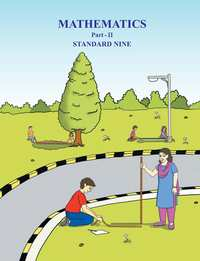## Chapter 3: Triangles

Practice Set 3.1Practice Set 3.2Practice Set 3.3Practice Set 3.4Practice Set 3.5Problem Set 3

#### Chapter 3: Triangles Exercise Practice Set 3.1 solutions [Pages 27 - 28]

Practice Set 3.1 | Q 1 | Page 27

In the given figure, ∠ ACD is an exterior angle of  Δ ABC . ∠ B = 40°, ∠ A = 70°.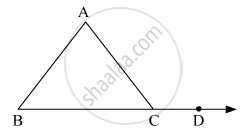Find the measure of ∠ ACD.

Practice Set 3.1 | Q 2 | Page 27

In Δ PQR, ∠ P = 70°, ∠ Q = 65 ° then find  ∠ R.

Practice Set 3.1 | Q 3 | Page 27

The measures of angles of a triangle are x°, ( x - 20)°, (x - 40)°. Find the measure of each angle.

Practice Set 3.1 | Q 4 | Page 27

The measure of one of the angles of a triangle is twice   the measure of its smallest angle and the measure of the other is thrice the measure of the smallest angle. Find the measures of the three angles.

Practice Set 3.1 | Q 5 | Page 28

In the given figure, measures of some angles are given. Using the measures find the values of x, y, z.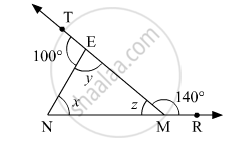Practice Set 3.1 | Q 6 | Page 28

In the given figure, line AB || line DE. Find the measures of ∠ DRE and ∠ ARE using given measures of some angles.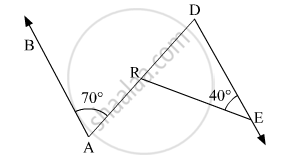Practice Set 3.1 | Q 7 | Page 28

In Δ ABC, bisectors of ∠ A and ∠ B intersect at point O. If ∠ C = 70° . Find measure of ∠ AOB.

Practice Set 3.1 | Q 8 | Page 28

In the given Figure, line AB || line CD and line PQ is the transversal. Ray PT and ray QT are bisectors of ∠ BPQ and ∠ PQD respectively.
Prove that m ∠ PTQ = 90 °.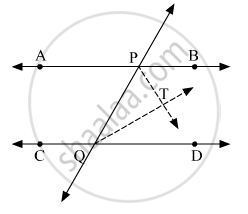Practice Set 3.1 | Q 9 | Page 28

Using the information shown in figure, find the measures of ∠ a, ∠ b and ∠ c.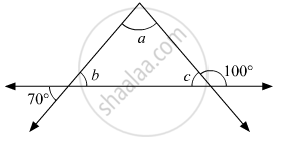Practice Set 3.1 | Q 10 | Page 28

In the given figure, line DE ||   line GF ray EG and ray FG are bisectors of ∠ DEF and ∠ DFM respectively. Prove that,

1. angle DEG = 1/2  angle EDF

2. EF = FG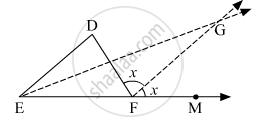#### Chapter 3: Triangles Exercise Practice Set 3.2 solutions [Pages 31 - 33]

Practice Set 3.2 | Q 1.1 | Page 31

In the following  example , a pair of triangles is shown. Equal parts of triangles in each pair are marked with the same sign. Observe the figure and state the test by which the triangle in each pair are congruent.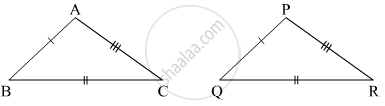By . . . . . . . . . . test
Δ ABC  ≅  ΔPQR

Practice Set 3.2 | Q 1.2 | Page 31

In the following example , a pair of triangles is shown. Equal parts of triangle in each pair are marked with the same sign. Observe the figure and state the test by which the triangle in each pair are congruent.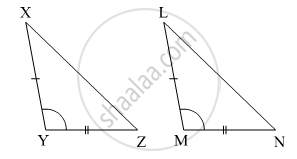By . . . . . . . . . . test
Δ XYZ  ≅  Δ LMN

Practice Set 3.2 | Q 1.3 | Page 31

In the following example , a pair of triangles is shown. Equal parts of triangle in each pair are marked with the same sign. Observe the figure and state the test by which the triangles in each pair are congruent.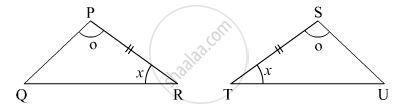By . . . . . . . . . . test
Δ LMN  ≅  Δ PTR

Practice Set 3.2 | Q 1.4 | Page 31

In the following example ,a pair of triangles is shown. Equal parts of triangle in each pair are marked with the same sign. Observe the figure and state the test by which the triangle in each pair are congruent.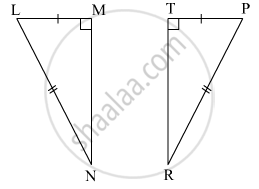By . . . . . . . . . . test
Δ LMN  ≅  Δ  PTR

Practice Set 3.2 | Q 2.1 | Page 32
Observe the information shown in pair of triangle given below. State the test by which the two triangles are congruent. Write the remaining congruent parts of the triangles.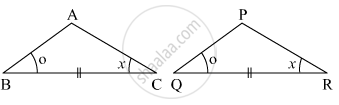From the information shown in the figure,
in Δ ABC and Δ PQR
∠ ABC  ≅  ∠PQR
seg BC ≅ seg QR
∠ACB  ≅ ∠PRQ
∴ Δ ABC ≅ Δ PQR ......    _________ test
∴ ∠ BAC ≅  ________    ..... corresponding angles of congruent triangles.
seg AB  ≅  ___ and  ___ ≅ seg PR } ..correspoding sides of congruent triangles
Practice Set 3.2 | Q 2.2 | Page 32

Observe the information shown in pair of triangle given below. State the test by which the two triangles are congruent. Write the remaining congruent parts of the triangles.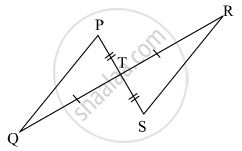From the information shown in the figure,

in ΔPTQ and Δ STR

seg PT ≅  seg ST

∠ PTQ  ≅ ∠ STR   ....Vertically opposite angles

∴ Δ PTQ  ≅ Δ STR  .... _____ test

∴ ∠ TPQ ≅ _____  and  ____ ≅ ∠ TRS  .....corresponding angles of congruent triangles

seg PQ ≅ ______ corresponding sides of congruent triangles

Practice Set 3.2 | Q 3 | Page 32

From the information shown in the figure, state the test assuring the congruence of Δ  ABC and Δ  PQR. Write the remaining congruent parts of the triangles.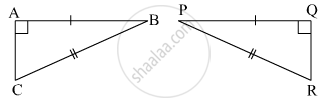Practice Set 3.2 | Q 4 | Page 32

As shown in the following figure, in  Δ LMN and Δ PNM, LM = PN, LN = PM. Write the test which assures the congruence of the two triangles. Write their remaining congruent parts.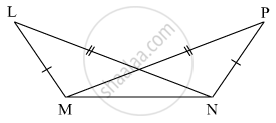Practice Set 3.2 | Q 5 | Page 32

In the given figure, seg AB ≅ seg CB and seg AD ≅ seg CD.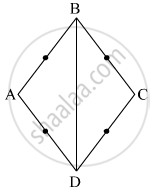Prove that Δ ABD ≅ Δ CBD

Practice Set 3.2 | Q 6 | Page 33

In the given figure, ∠ P ≅ ∠R seg PQ ≅ seg RQ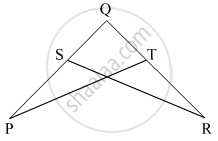Prove that, Δ PQT ≅ Δ RQS

#### Chapter 3: Triangles Exercise Practice Set 3.3 solutions [Page 38]

Practice Set 3.3 | Q 1 | Page 38

Find the values of x and y using the information shown in figure .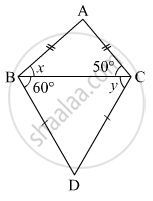Find the measure of ∠ ABD and m∠ ACD.

Practice Set 3.3 | Q 2 | Page 38

The length of hypotenuse of a right angled triangle is 15. Find the length of median of its hypotenuse.

Practice Set 3.3 | Q 3 | Page 38

In Δ PQR, ∠ Q = 90° , PQ = 12, QR = 5 and QS is a median. Find l(QS).

Practice Set 3.3 | Q 4 | Page 38

In the given figure, P point G is the point of concurrence of the medians of Δ  PQR . If GT = 2.5, find the lengths of PG and PT.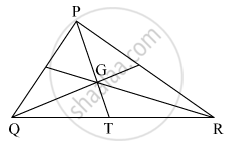#### Chapter 3: Triangles Exercise Practice Set 3.4 solutions [Pages 43 - 44]

Practice Set 3.4 | Q 1 | Page 43

In the given figure, point A is on the bisector of ∠ XYZ. If AX = 2 cm then find AZ.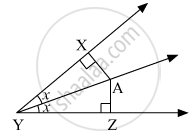Practice Set 3.4 | Q 2 | Page 43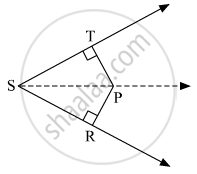In the given figure, ∠ RST = 56° , seg PT ⊥ ray ST, seg PR ⊥  ray SR and seg PR ≅  seg PT Find the measure of ∠ RSP. State the reason for your answer.

Practice Set 3.4 | Q 3 | Page 43

In Δ PQR, PQ = 10 cm, QR = 12 cm, PR = 8 cm. Find out the greatest and the smallest angle of the triangle.

Practice Set 3.4 | Q 4 | Page 43

In Δ FAN, ∠ F = 80° , ∠ A = 40° . Find out the greatest and the smallest side of the triangle. State the reason.

Practice Set 3.4 | Q 5 | Page 43

Prove that an equilateral triangle is equiangular.

Practice Set 3.4 | Q 6 | Page 44

Prove that, if the bisector of ∠ BAC of Δ ABC is perpendicular to side BC, then Δ ABC is an isosceles triangle.

Practice Set 3.4 | Q 7 | Page 44

In the given figure, if seg PR ≅ seg PQ, show that seg PS > seg PQ.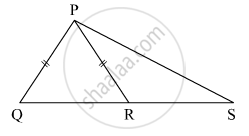Practice Set 3.4 | Q 8 | Page 44

In the given figure, in Δ ABC, seg AD and seg BE are altitudes and AE = BD. Prove that seg AD ≅ seg BE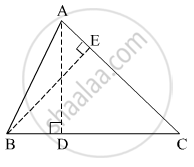#### Chapter 3: Triangles Exercise Practice Set 3.5 solutions [Page 47]

Practice Set 3.5 | Q 1 | Page 47

If Δ  XYZ ~  Δ  LMN, write the corresponding angles of the two triangles and also write the ratios of corresponding sides.

Practice Set 3.5 | Q 2 | Page 47

In Δ  XYZ, XY = 4 cm, YZ = 6 cm, XZ = 5 cm, If  Δ  XYZ ~
Δ​ PQR  and PQ = 8 cm then find the lengths of remaining sides of Δ PQR.

Practice Set 3.5 | Q 3 | Page 47

Draw a sketch of a pair of similar triangles. Label them. Show their corresponding angles by the same signs. Show the lengths of corresponding sides by numbers in proportion.

#### Chapter 3: Triangles Exercise Problem Set 3 solutions [Pages 49 - 50]

Problem Set 3 | Q 1.1 | Page 49

Choose the correct alternative answer for the following question.

If two sides of a triangle are 5 cm and 1.5 cm, the lenght of its third side cannot be . . . . . . . .

• 3.7 cm

• 4.1 cm

• 3.8 cm

•  3.4 cm

Problem Set 3 | Q 1.2 | Page 49

Choose the correct alternative answer for the following question.

In Δ PQR, If ∠ R > ∠ Q then . . . . . . . .

• QR > PR

• PQ > PR

• PQ < PR

• QR < PR

Problem Set 3 | Q 1.3 | Page 49

In Δ TPQ, ∠  T = 65° , ∠ P = 95° which of the following is a true statement ?

• PQ < TP

• PQ < TQ

• TQ < TP < PQ

• PQ < TP < TQ

Problem Set 3 | Q 2 | Page 49

Δ ABC is isosceles in which AB = AC. Seg BD and seg CE are medians. Show that BD = CE.

Problem Set 3 | Q 3 | Page 49

In Δ PQR, If PQ > PR and bisectors of ∠ Q and ∠ R intersect at S. Show that SQ > SR.Problem Set 3 | Q 4 | Page 49

In the figure, point D and E are on side BC of Δ ABC, such that BD = CE and AD = AE.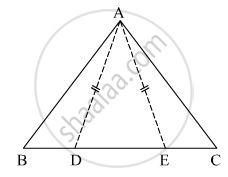Show that Δ ABD ≅ Δ ACE.

Problem Set 3 | Q 5 | Page 49

In the given figure, point S is any point on side QR of Δ PQR Prove that : PQ + QR + RP > 2PS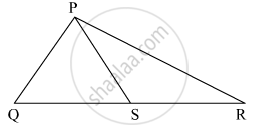Problem Set 3 | Q 6 | Page 50

In the given figure, bisector of  ∠ BAC intersects side BC at point D. Prove that AB > BD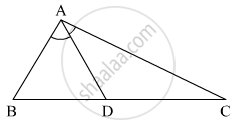Problem Set 3 | Q 7 | Page 50

In the given figure, seg PT is the bisector of ∠ QPR. A line through R intersects ray QP at point S. Prove that PS = PR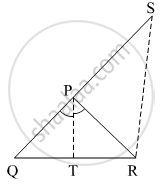Problem Set 3 | Q 8 | Page 50

In the given figure, seg AD ⊥  seg BC. seg AE  is the bisector of ∠ CAB and C - E - D. Prove that

∠DAE = 1/2 (∠C - ∠B)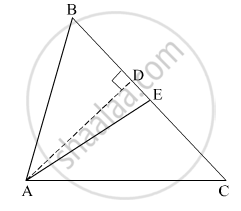## Chapter 3: Triangles

Practice Set 3.1Practice Set 3.2Practice Set 3.3Practice Set 3.4Practice Set 3.5Problem Set 3

#### Balbharati Balbharati Class 9 Mathematics 2 Geometry## Balbharati solutions for Class 9 Geometry chapter 3 - Triangles

Balbharati solutions for Class 9 chapter 3 (Triangles) include all questions with solution and detail explanation. This will clear students doubts about any question and improve application skills while preparing for board exams. The detailed, step-by-step solutions will help you understand the concepts better and clear your confusions, if any. Shaalaa.com has the Maharashtra State Board Balbharati Class 9 Mathematics 2 Geometry solutions in a manner that help students grasp basic concepts better and faster.

Further, we at Shaalaa.com provide such solutions so that students can prepare for written exams. Balbharati textbook solutions can be a core help for self-study and acts as a perfect self-help guidance for students.

Concepts covered in Class 9 Geometry chapter 3 Triangles are Similar Triangle, Properties of inequalities of sides and angles of a triangle, Angle bisector theorem, Perpendicular bisector Theorem, Median of a Triangle, Property of 30-60-90 Triangle Theorem, Isoscles Triangle Theorem, Congruence of Triangles, Theorem of remote interior angles of a triangle, Concept of Triangles.

Using Balbharati Class 9 solutions Triangles exercise by students are an easy way to prepare for the exams, as they involve solutions arranged chapter-wise also page wise. The questions involved in Balbharati Solutions are important questions that can be asked in the final exam. Maximum students of Maharashtra State Board Class 9 prefer Balbharati Textbook Solutions to score more in exam.

Get the free view of chapter 3 Triangles Class 9 extra questions for and can use Shaalaa.com to keep it handy for your exam preparation

S